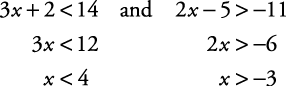## Compound Inequalities

A compound inequality is a sentence with two inequality statements joined either by the word “or” or by the word “and.” “And” indicates that both statements of the compound sentence are true at the same time. It is the overlap or intersection of the solution sets for the individual statements. “Or” indicates that, as long as either statement is true, the entire compound sentence is true. It is the combination or union of the solution sets for the individual statements. A compound inequality that uses the word “and” is known as a conjunction. Although “and” and “or” are parts of speech known as conjunctions, the mathematical conjunction has a different meaning from the grammatical one. To prove the point, the conjunction (part of speech) “or”—when used in a compound inequality—forms what is known as a disjunction. Just remember “con” means “with another,” and “dis” means “one OR the other.”

##### Example 1

Solve for x: 3 x + 2 < 14 and 2 x – 5 > –11.

Solve each inequality separately. Since the joining word is “and,” this indicates that the overlap or intersection is the desired result.x < 4 indicates all the numbers to the left of 4, and x > –3 indicates all the numbers to the right of –3. The intersection of these two graphs is all the numbers between –3 and 4. The solution set is

{ x| x > –3 and x < 4}

Another way this solution set could be expressed is

{ x|–3 < x < 4}

When a compound inequality is written without the expressed word “and” or “or,” it is understood to automatically be the word “and.” Reading { x|–3 < x < 4} from the “ x” position, you say (reading to the left), “ x is greater than –3 and (reading to the right) x is less than 4.” The graph of the solution set is shown in Figure 1.